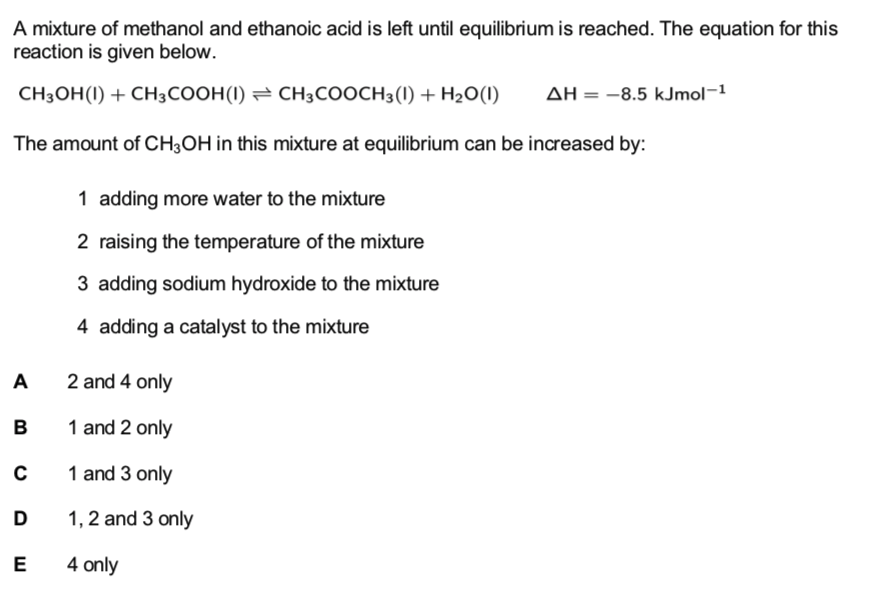# A mixture of methanol and ethanoic acid is left until equilibrium is reached. The equation for this reaction is given below. CH3OH(l) + CH3COOH(l) ⇌ CH3COOCH3(l) + H2O(l) ΔH = -8.5kJmol^-1 The amount of CH3OH in this mixture at equilibrium can be increased by: 1. adding more water to the mixture. 2. raising the temperature of the mixture. 3. adding sodium hydroxide to the mixture 4. adding a catalyst to the mixture